# Know How to Build a Resistor/Capacitor Selection Box

In order to design a circuit and to try with various values of capacitors and resistors you would switch with different electronic components for the correct combination to suit your requirements. It will become difficult to determine what resistance and capacitance you want to get the filtering attributes.  With a Selection box as shown in the above gives many values by just turning the knob which can test many different values.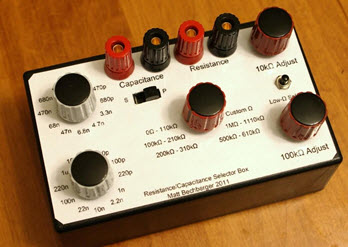Resistor/Capacitor Selection Box

Features of Resistor/Capacitor Selection Box: For the precise resistances, 10 turn potentiometers are needed,Wire terminals, Protection button with low resistance, Orientation switch for Series or Parallel capacitors, Twenty-two capacitors on rotary switches. The necessary materials with calculated values for all possible combinations of capacitors are used in this selection box.

## Steps to Build a Resistor/Capacitor Selection Box

To design a resistor/capacitor selection box mainly, the following steps include

#### Required  Materials

4x Binding posts, 2x 1 Pole 12 Throw rotary switches, 1 Pole 6 Throw a rotary switch, 10k Pot (multi-turn is best for increased accuracy), 100k Pot (multi-turn optional), DPDT slide switch, 2x 100k 1% resistors, 3x 200k 1% resistors, 1M 1% resistor, 4.5″ x 6″ x 3″ project box, 5x Knobs, Solder, Ribbon cable, Capacitors:

#### Required Tools

Drill and various bits, Wrench, Hot glue gun, Soldering Iron, Phillips Screwdriver, Tin snips, Printer, Square needle file, Center punch, Tape and Scissors

### Schematic  Diagram of Resistor/Capacitor Selection Box

The schematic diagram of resistor ,capacitor selection box comprises of two separate parts.They are the resistance portion and the capacitance portion.  The capacitance portion consists of two variable capacitors consisting of a rotary switch and also 11 capacitors each.  The DPDT toggle permits those to move from a parallel to series configuration wherever required in order to obtain more combinational values.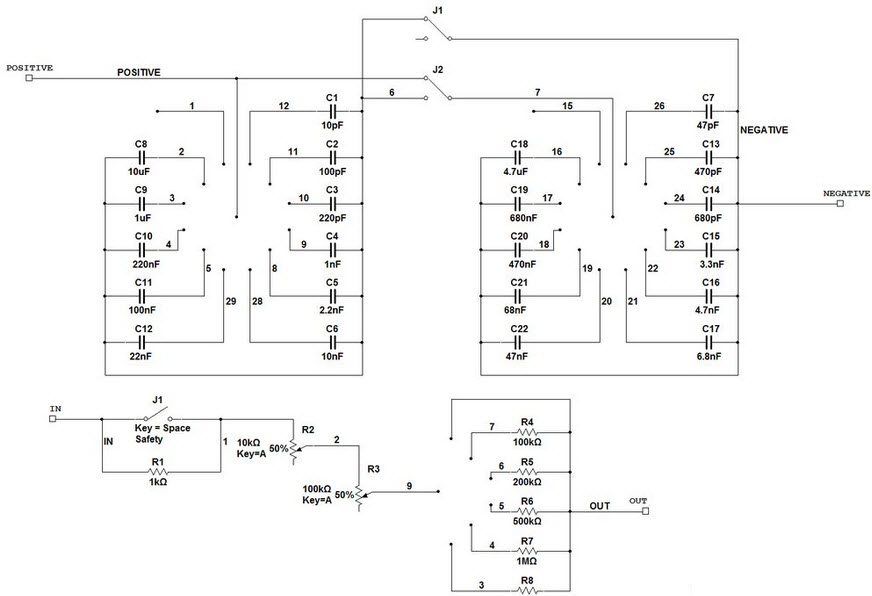Schematic and Template

The resistance portion has1k ohm resistor on a button which behaves like a low-ohm and if not pressed total resistance would not go below 1000 ohms, a rotary switch for extra resistance choices and two potentiometers.

### Template Design and Drilling

The dimensions for the designing of template and drilling are 4.5” by 6. To place the template in  the box, print it out first, then cut the borders. Tape the template on the top of the enclosure and use the center punch through the black holes on the template. Take out the template and drill hole in each spot using a 1/8 bit. Measure the diameter of the potentiometers and switches, and drill fittingly sized holes in the suitable holes.For the switch, using a bit, drill 2 holes the width of the black square on the template, then use a square-shaped file to eliminate the remaining material.

### Assembly and Cabling

To design a simple, durable and cheap template, print a copy and laminate it. Cut the edges to the proper shape and hold the enclose up in the air with the template on the front of the enclosure. And check into the backside of the enclosure with a light in front. This front light is used to line up the holes to the midpoint of the holes where you drilled for the parts and tape it in place. Take a knife and cut into every hole to remove the laminated paper, that covers the hole in the plastic.Insert the components in each hole and tighten the nuts. The switch is held in place with hot glue.Meanwhile the caps for each switch are connected together by their negative leads and solder the negative leads in a column.

#### Resistor

Resistor is defined as an electrical component which decreases electric current in the circuit. The capability of a resistor to reduce the current is known as resistance. The units of the resistor is  ohms and the symbol  is Ω.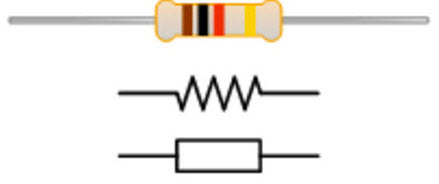Resistor

The main aim of a resistor within an electrical or electronic circuit is to regulate or to adjust the flow of electrons through the circuit. Resistors are connected together in different series and parallel combinations to form resistor networks, which can act as voltage droppers, voltage dividers or current limiters within a circuit. Resistors are Passive Devices without any power source, but attenuate or decrease the voltage or flow of current. This type of transmission of electrical energy will be lost in the form of heat.

#### Ohm’s law

Ohms law states that, dessipation due to the resistance

Where V in volts (V), I in amps (A), R in ohms (Ω)
I = V/R

Power consumption P in watts (W) is equal to the current of resistor I in amps (A) times the voltage V of resistor in volts (V)
P = I × V

The resistor’s power consumption P in watts (W) is equal to the square value of the resistor’s current I in amps (A) times the resistor’s resistance R in ohms (Ω):

P = I 2 × R

The resistor’s power consumption P in watts (W) is equal to the square value of the resistor’s voltage V in volts (V) divided by the resistor’s resistance R in ohms (Ω):

P = V 2 / R

The total equivalent resistance of resistors in series Rtotal is the sum of the resistance values:
Rtotal = R1+ R2+ R3+…

#### Capacitor

A capacitor consists of two conducting plates separated by an insulating material called the dielectric. A capacitor is a passive electronic component which stores energy in the form of an electrostatic field.  The capacitance is directly proportional to the surface areas of the plates and inversely proportional to the separation between the plates. Capacitance also depends on the dielectric constant of the substance separating the plates. Capacitors can be fabricated onto integrated circuit (IC) chips. Farad is the unit of capacitance.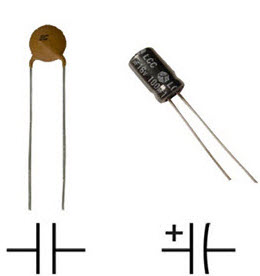Capacitor

#### Capacitance

Capacitance is defined as the capability of an object to store an electrical charge. Any substance which can be electrically charged shows capacitance. Any form of energy storage device is a parallel-plate capacitor. In a parallel plate capacitor, capacitance is directly proportional to the surface area of the conductor plates and inversely proportional to the separation distance between the plates. If the charges on the plates are +q and −q respectively, and V gives the voltage between the plates, then the capacitance C is given by

Capacitance C = q/v

that gives voltage/current relationship

A resistor–capacitor circuit or RC circuit or RC filter or RC network is an electric circuit comprising of resistors and capacitors run by current source or voltage. The first order RC circuit consists of one resistor and one capacitor and it will be the simplest type of RC circuit.

RC circuits can be used to filter a signal by blocking certain frequencies and passing others. The two most common RC filters are the high-pass filters, band-pass filters, low-pass filters and band-stop filters which needs RLC filters.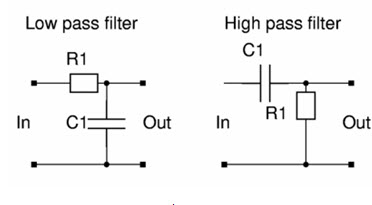RC Filter Circuit

#### Arduino Based Underground able Fault Detection

The objective of this project is to determine the distance of underground cable fault from the base station in kilometers Using An Arduino board. The underground cable system is a common practice followed in many urban areas. While a fault occurs for some reason, at that time the repairing process related to that particular cable is difficult due to not knowing the exact location of the cable fault.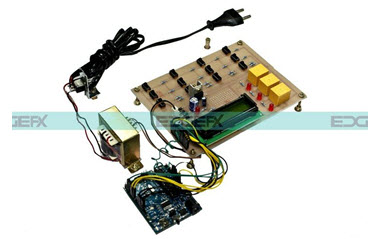Arduino Based Underground Cable Fault Detection Project kit by Edgefxkits.com

The proposed system is to find the exact location of the fault. The project uses the standard concept of Ohms law,  i.e., when a low DC voltage is applied at the feeder end through a series resistor (Cable lines), then the current would vary depending upon the location of a fault in the cable. In case there is a short circuit (Line to Ground), the voltage across series resistor changes accordingly, which is then fed to the inbuilt ADC of Arduino board to develop precise digital data for display in kilometers.

This  project is designed with a set of resistors representing cable length in KM’s and fault creation is made by a set of switches at every known KM to cross check the accuracy of the same. The fault occurring at a particular distance and the respective phase is displayed on an LCD interfaced to the Arduino board. Further, this project can be enhanced by using a capacitor in an AC circuit to measure the impedance which can even locate the open circuited cable, unlike the short circuited fault only using resistors in  a DC circuit as followed in the above proposed project.

Therefore, this is all about how to build a resistor.capacitor selection box, and its applications.We believe that you have got a better idea about this article.Furthermore, any doubts regarding this or electronics projects you can contact us by commenting in the comment section below.

Photo Credits: